Test: Power Electronics- 6

# Test: Power Electronics- 6

Test Description

## 10 Questions MCQ Test GATE Electrical Engineering (EE) 2023 Mock Test Series | Test: Power Electronics- 6

Test: Power Electronics- 6 for Electrical Engineering (EE) 2023 is part of GATE Electrical Engineering (EE) 2023 Mock Test Series preparation. The Test: Power Electronics- 6 questions and answers have been prepared according to the Electrical Engineering (EE) exam syllabus.The Test: Power Electronics- 6 MCQs are made for Electrical Engineering (EE) 2023 Exam. Find important definitions, questions, notes, meanings, examples, exercises, MCQs and online tests for Test: Power Electronics- 6 below.
Solutions of Test: Power Electronics- 6 questions in English are available as part of our GATE Electrical Engineering (EE) 2023 Mock Test Series for Electrical Engineering (EE) & Test: Power Electronics- 6 solutions in Hindi for GATE Electrical Engineering (EE) 2023 Mock Test Series course. Download more important topics, notes, lectures and mock test series for Electrical Engineering (EE) Exam by signing up for free. Attempt Test: Power Electronics- 6 | 10 questions in 30 minutes | Mock test for Electrical Engineering (EE) preparation | Free important questions MCQ to study GATE Electrical Engineering (EE) 2023 Mock Test Series for Electrical Engineering (EE) Exam | Download free PDF with solutions
 1 Crore+ students have signed up on EduRev. Have you?
Test: Power Electronics- 6 - Question 1

### A single-phase voltage source square-wave inverter feeds pure inductive load. The waveform of the load current will be

Detailed Solution for Test: Power Electronics- 6 - Question 1

A single-phase voltage source square-wave inverter feeds pure inductive load. The waveform of the load current will be triangular.

*Answer can only contain numeric values
Test: Power Electronics- 6 - Question 2

### The output value e1 and e2 of two full bridge inverter are added using output transformer. In order to eliminate the 3rd harmonic from output voltage, the phase angle between e1 and e2 should be ______ degree.

Detailed Solution for Test: Power Electronics- 6 - Question 2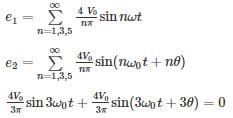It is possible when

3θ = π

θ = π/3

Test: Power Electronics- 6 - Question 3

### A single phase full bridge inverter is fed from a 48 V battery and is delivering power to a pure resistance load what is the value of fundaments output voltage?

Detailed Solution for Test: Power Electronics- 6 - Question 3

Concept:

For single phase full bridge inverter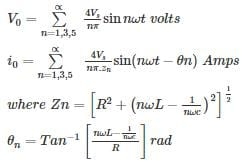Calculation:

The RMS value of the fundamental voltage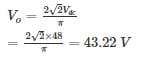*Answer can only contain numeric values
Test: Power Electronics- 6 - Question 4

A single phase full-bridge inverter, connected to 230 V dc source, feeds a resistive load 20 Ω find the average current of each SCR.

Detailed Solution for Test: Power Electronics- 6 - Question 4

Concept:

In full-bridge inverter SCR conducts for 180° during one cycle of 360°

Average SCR current =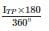and peak current of each thyristor =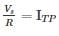Calculation:
ITP = 230/20 = 11.5A
∴ Average current of each SCR =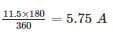Test: Power Electronics- 6 - Question 5

A single phase full bridge inverter feeds power to a load of R = 12 Ω and L = 0.04 H from a 400 V dc source. If the inverter operates the frequency of 50 Hz, determine the power delivered to load (in watt) for square wave operation. (Consider first and third harmonic).

Detailed Solution for Test: Power Electronics- 6 - Question 5

RMS value of fundamental voltage is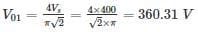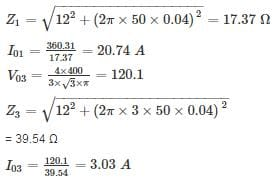RMS value of resultant.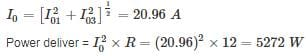*Answer can only contain numeric values
Test: Power Electronics- 6 - Question 6

A single phase full converter, connected 240 V, 50 Hz source, is feeding a load R = 10 Ω in series with a larger inductance that makes the load current ripple free. For a firing angle 30°, calculated R.M.S value of fundamental current (in A).

Detailed Solution for Test: Power Electronics- 6 - Question 6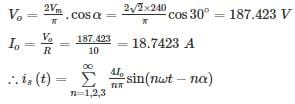The R.M.S value of nth harmonic input current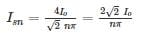R.M.S value of fundamental current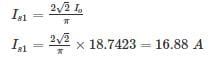*Answer can only contain numeric values
Test: Power Electronics- 6 - Question 7

A single phase half bridge inverter is feeding power into a load of resistance 20 Ω, dc input to the inverter is 500V. Then the power factor is_____

Detailed Solution for Test: Power Electronics- 6 - Question 7

RMS value of output voltage is,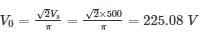RMS value of load current is,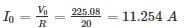Power delivered,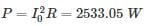Power factor, cosϕ = P/VI = 0.99

Test: Power Electronics- 6 - Question 8

A single phase half bridge VSI has load R = 1.8 Ω and DC source voltage 130 – 0 – 130 V. Find the power delivered to the load due to fundamental current.

Detailed Solution for Test: Power Electronics- 6 - Question 8

Vdc = 260 V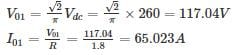P01 = (65.023)(1.8)

= 7610.38 W

= 7.61 kW

*Answer can only contain numeric values
Test: Power Electronics- 6 - Question 9

A single phase full converter feeds power to RLE load. If one of the four SCR gets open circuited due to fault. Find the value of average load current (in A) for firing angle 40°.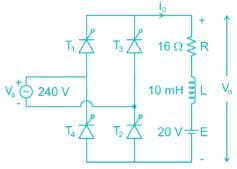Detailed Solution for Test: Power Electronics- 6 - Question 9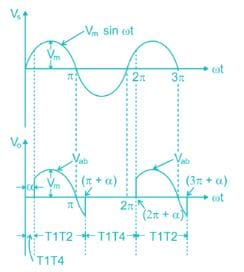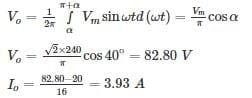Test: Power Electronics- 6 - Question 10

A single phase half-bridge inverter, connected to 230 V dc source, feeds a resistive load of 15 Ω.

The Input power factor is

Detailed Solution for Test: Power Electronics- 6 - Question 10

Inputpowerfactor =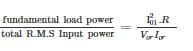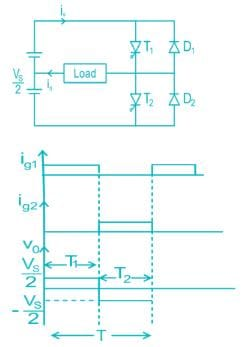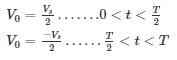Fourier series of output voltage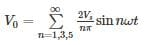RMS value of fundamental voltage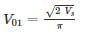R.M.S value of fundamental current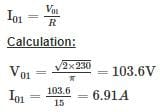Total R.M.S output voltage =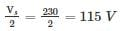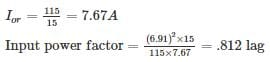## GATE Electrical Engineering (EE) 2023 Mock Test Series

22 docs|274 tests
 Use Code STAYHOME200 and get INR 200 additional OFF Use Coupon Code
Information about Test: Power Electronics- 6 Page
In this test you can find the Exam questions for Test: Power Electronics- 6 solved & explained in the simplest way possible. Besides giving Questions and answers for Test: Power Electronics- 6, EduRev gives you an ample number of Online tests for practice

## GATE Electrical Engineering (EE) 2023 Mock Test Series

22 docs|274 tests